# Chapter 41 Different Ways of Plotting U.S. Map in R

Zhiyi Guo and Fan Wu

## 41.1 Introduction

When dealing with national data/geographical data, i.e., election results, it is often useful to visualize the data onto a map as it could help draw conclusion geographycally. Hence, in this tutorial, we will explore different packages that could help with mapping geographical data, specifically for the U.S.

## 41.2 Using `usmap` package

This is probabily the most convinent package to plot a U.S. map along with some data. usmap provides very helpful functions to select certain regions within the U.S.. Another feature of this package is that it creates a ggplot object and hence we could use all the nice functions that come with ggplot2 package.

Package(s) we need:

``````#install.packages("usmap")
library(usmap) #import the package
library(ggplot2) #use ggplot2 to add layer for visualization``````

Plot all states of the U.S. to create an empty map.

``````plot_usmap(regions = "states") +
labs(title = "U.S. States",
subtitle = "This is a blank map of the United States.") +
theme(panel.background=element_blank())``````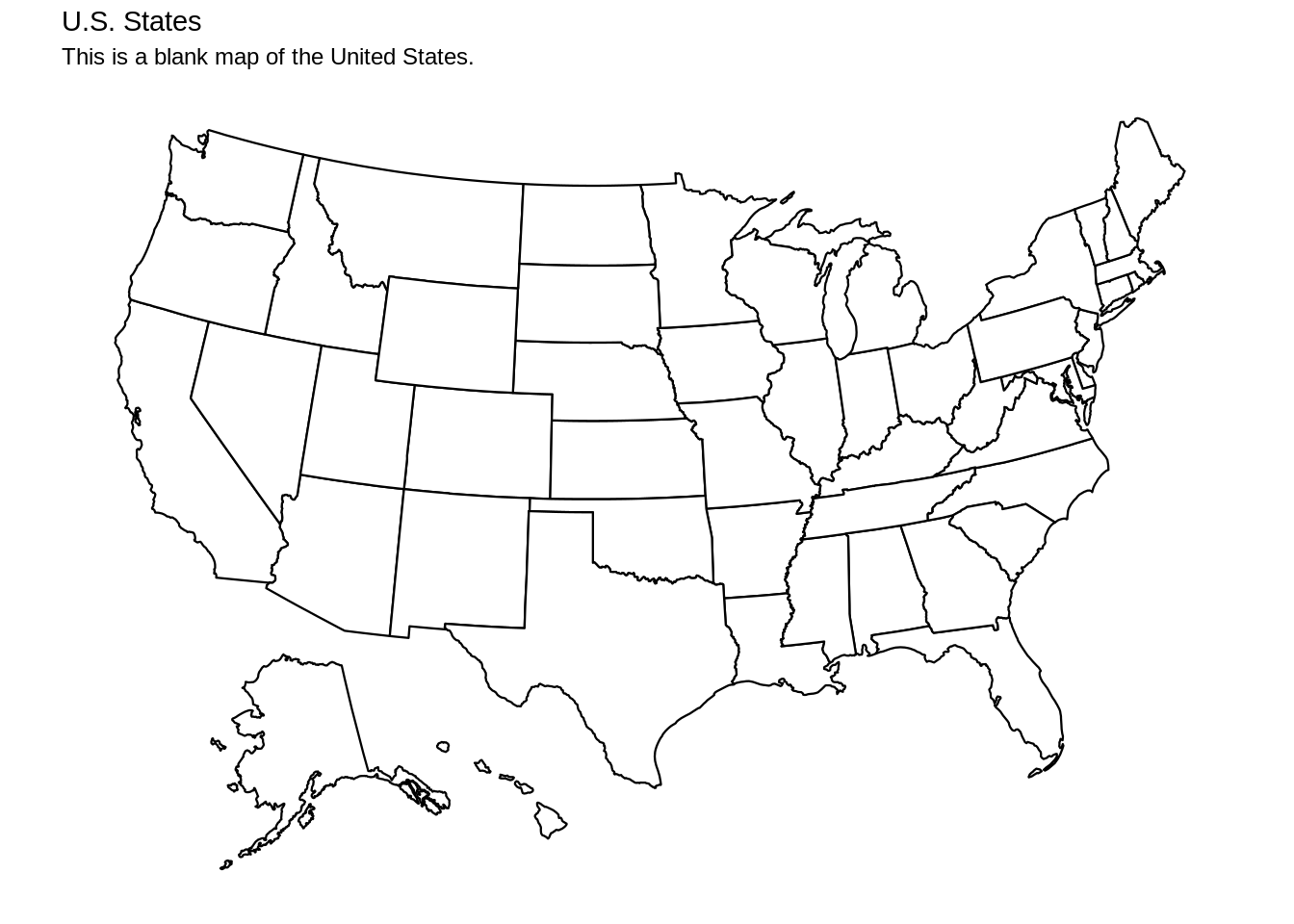Besides states, we could also plot all counties of the U.S.

``````plot_usmap(regions = "counties") +
labs(title = "U.S. counties",
subtitle = "This is a blank map of the United States.") +
theme(panel.background=element_blank())``````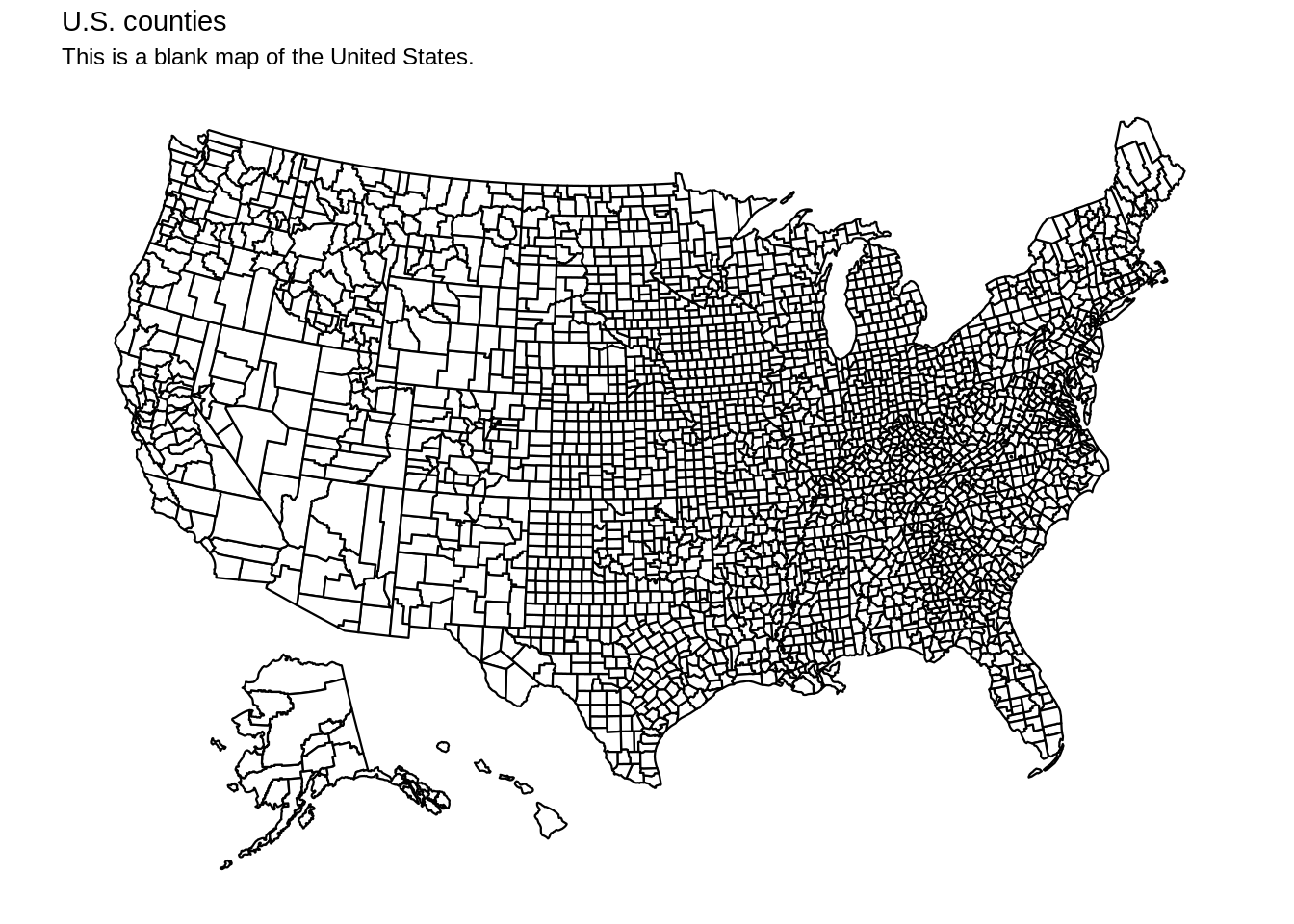usmap also provides many different regions to select, for example, the South region. The “include” and “exclude” functions give us freedom to select/delete regions as we desire, and this works for states and counties.

``plot_usmap(include = .south_region, exclude = c("VA"), labels = TRUE)``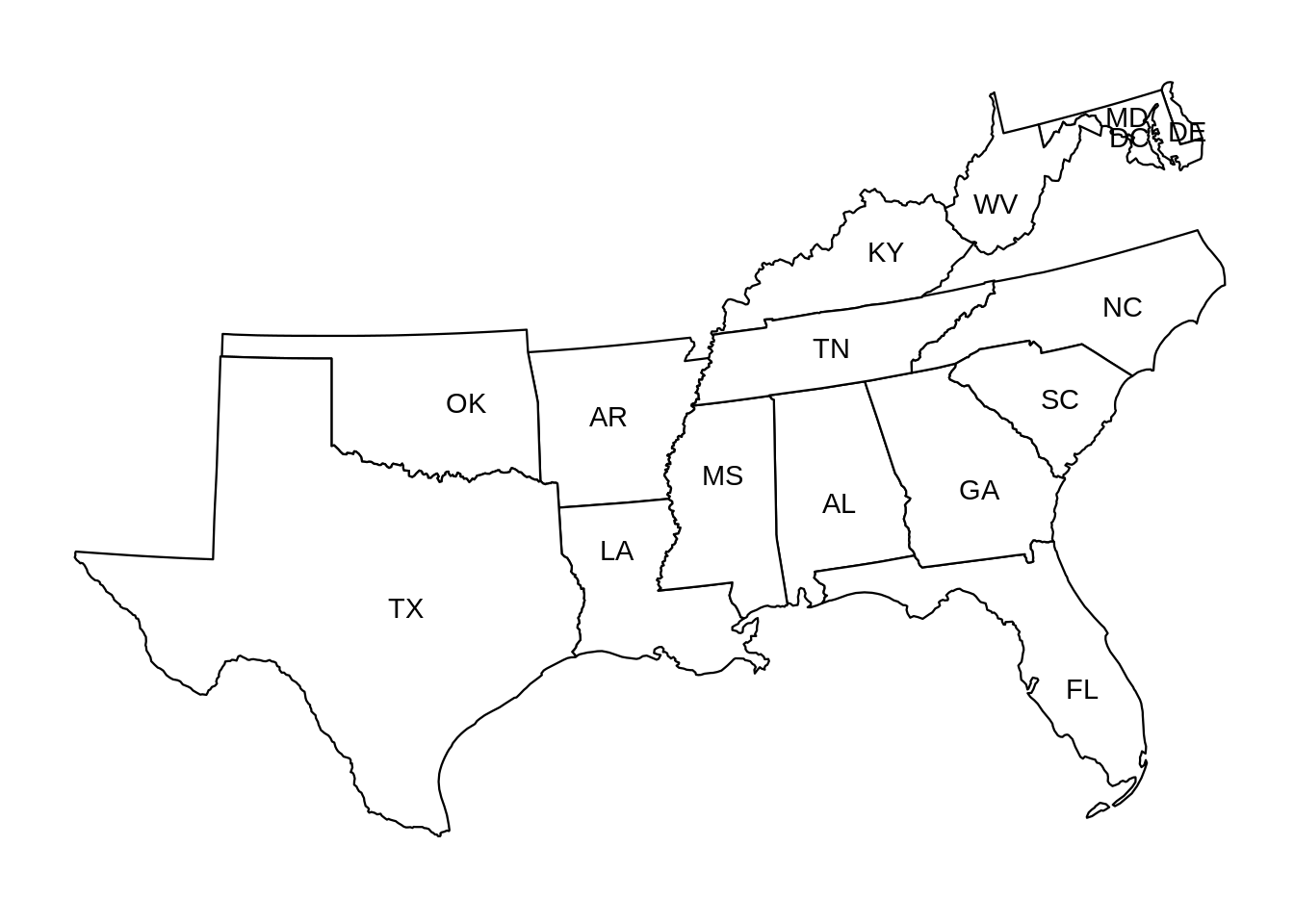You could also select certain states using factors(state abbreviations).

``````plot_usmap(include = c("CT", "ME", "MA", "NH", "VT")) +
labs(title = "New England Region") +
theme(panel.background = element_rect(color = "blue"))``````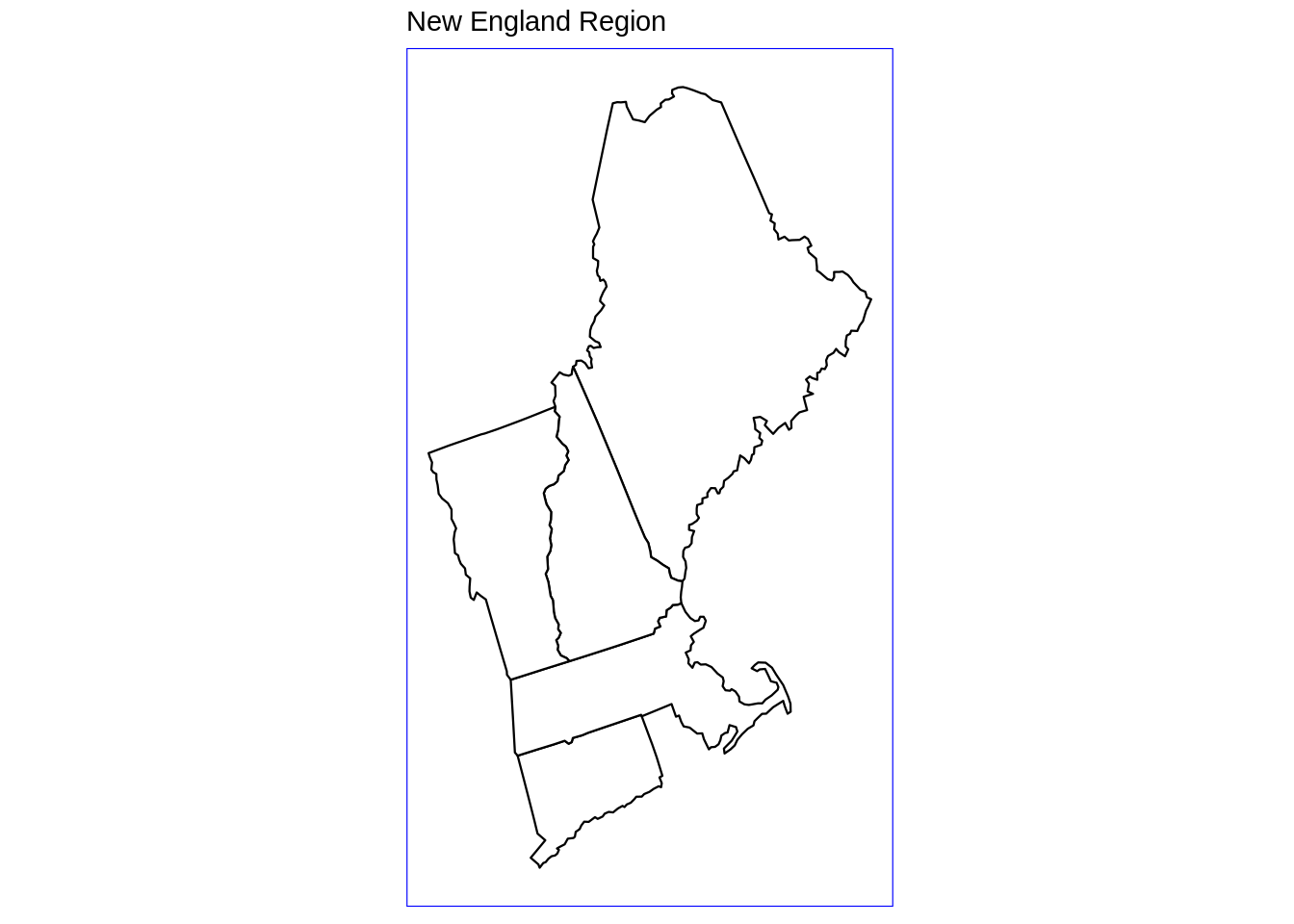The complete list of regions to choose is the following: .east_north_central: East North Central census division .east_south_central: East South Central census division .midwest_region: Midwest census region .mid_atlantic: Mid-Atlantic census division .mountain: Mountain census division .new_england: New England census division .northeast_region: Northeast census region .north_central_region: North-Central census region .pacific: Pacific census division .south_atlantic: South Atlantic census division .south_region: South census region .west_north_central: West North Central census division .west_region: West census region .west_south_central: West South Central census division usmap really provides users with the choice to mix and match areas of interest, hence makes it very desirable to manipulate the map.

The package comes with geographical/national data sets such as population, poverty, and etc. For example, using countypov data set from the package, we could see the poverty estimates for New England counties in 2014:

``````plot_usmap(data = countypov, values = "pct_pov_2014", include = c("CT", "ME", "MA", "NH", "VT"), color = "blue") +
scale_fill_continuous(low = "white", high = "blue", name = "Poverty Percentage Estimates", label = scales::comma) +
labs(title = "New England Region", subtitle = "Poverty Percentage Estimates for New England Counties in 2014") +
theme(legend.position = "right")``````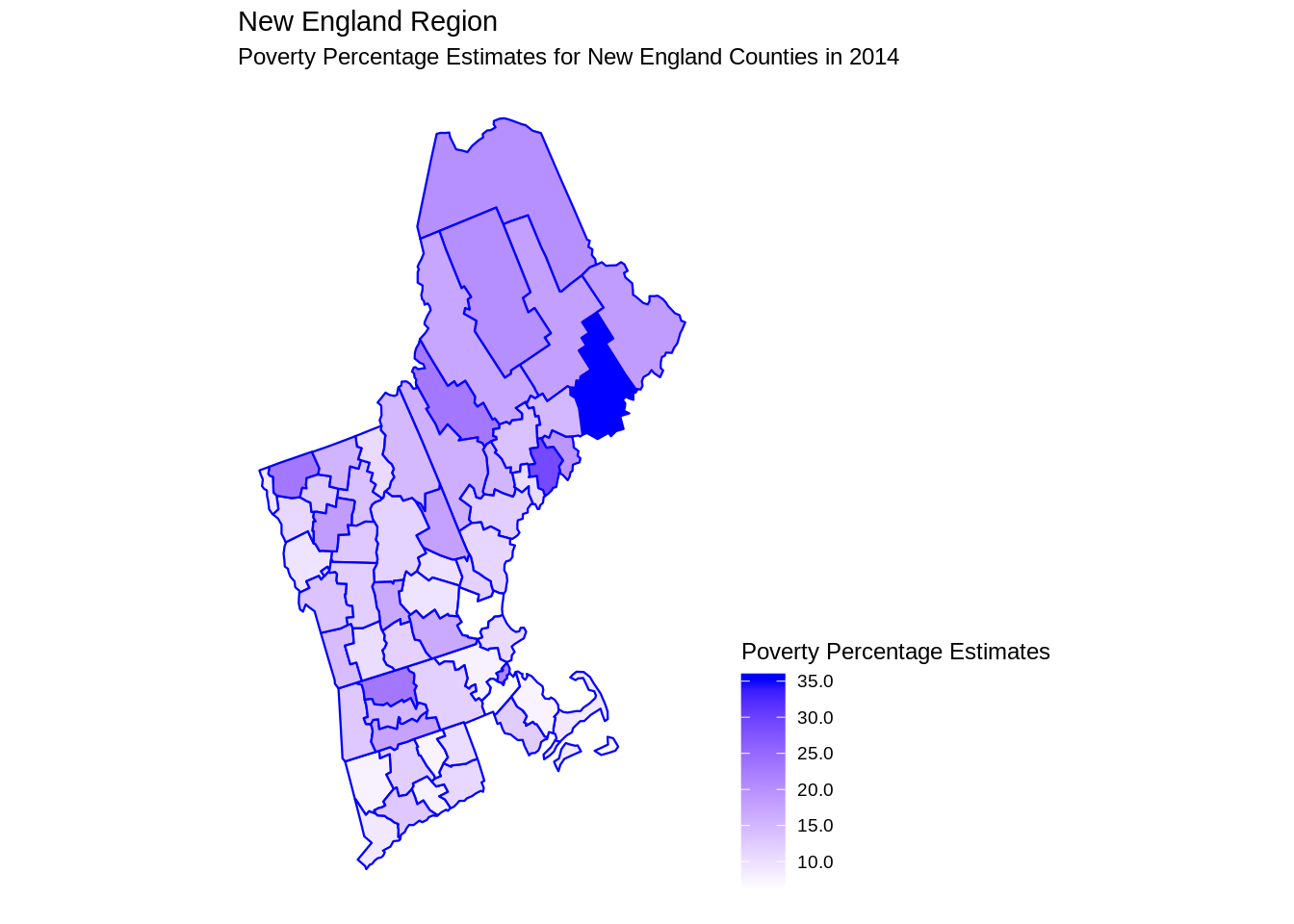We could also see population of these counties in 2015:

``````plot_usmap(data = countypop, values = "pop_2015", include = .new_england, color = "red") +
scale_fill_continuous(low = "white", high = "red", name = "Population", label = scales::comma) +
labs(title = "New England Region", subtitle = "Population in New England Counties in 2014") +
theme(legend.position = "right")``````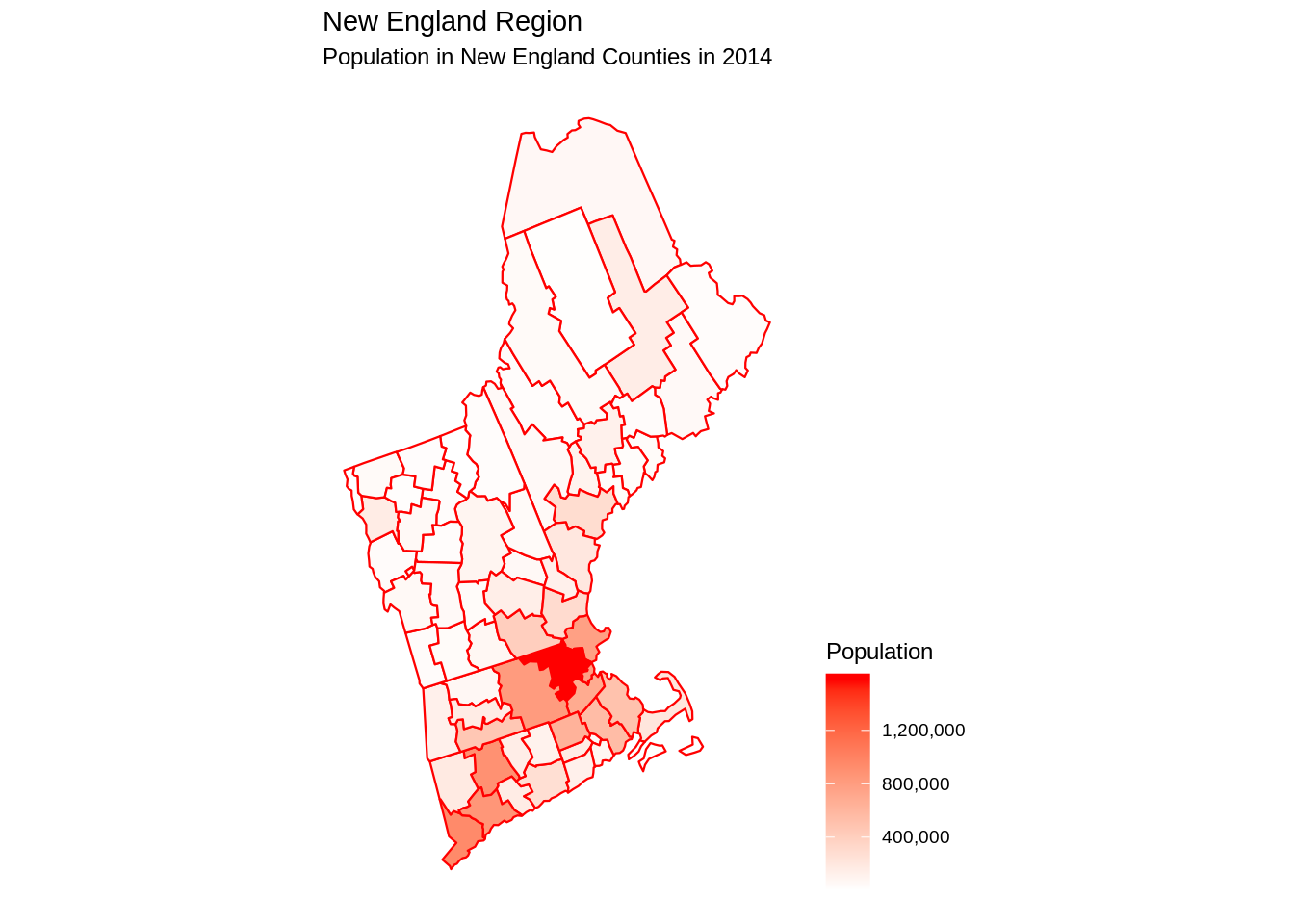## 41.3 Using `ggplot2` package

(Note: In this part, we only care about continental U.S.)

First, we get U.S. map data. In R, there are packages named `maps` and `mapdata` which save a lot of map information, for instance, continents, countries and states. We can use their data directly by using `map_data` function in `ggplot2` package.

Package(s) we need:

``````library(ggplot2)
library(maps)
library(mapdata)``````
``usa <- map_data('usa')``

Next, we use `geom_ploygon` function to plot U.S. map.

``````ggplot(data=usa, aes(x=long, y=lat, group=group)) +
geom_polygon(fill='lightblue') +
theme(axis.title.x=element_blank(), axis.text.x=element_blank(), axis.ticks.x=element_blank(),
axis.title.y=element_blank(), axis.text.y=element_blank(), axis.ticks.y=element_blank()) +
ggtitle('U.S. Map') +
coord_fixed(1.3)``````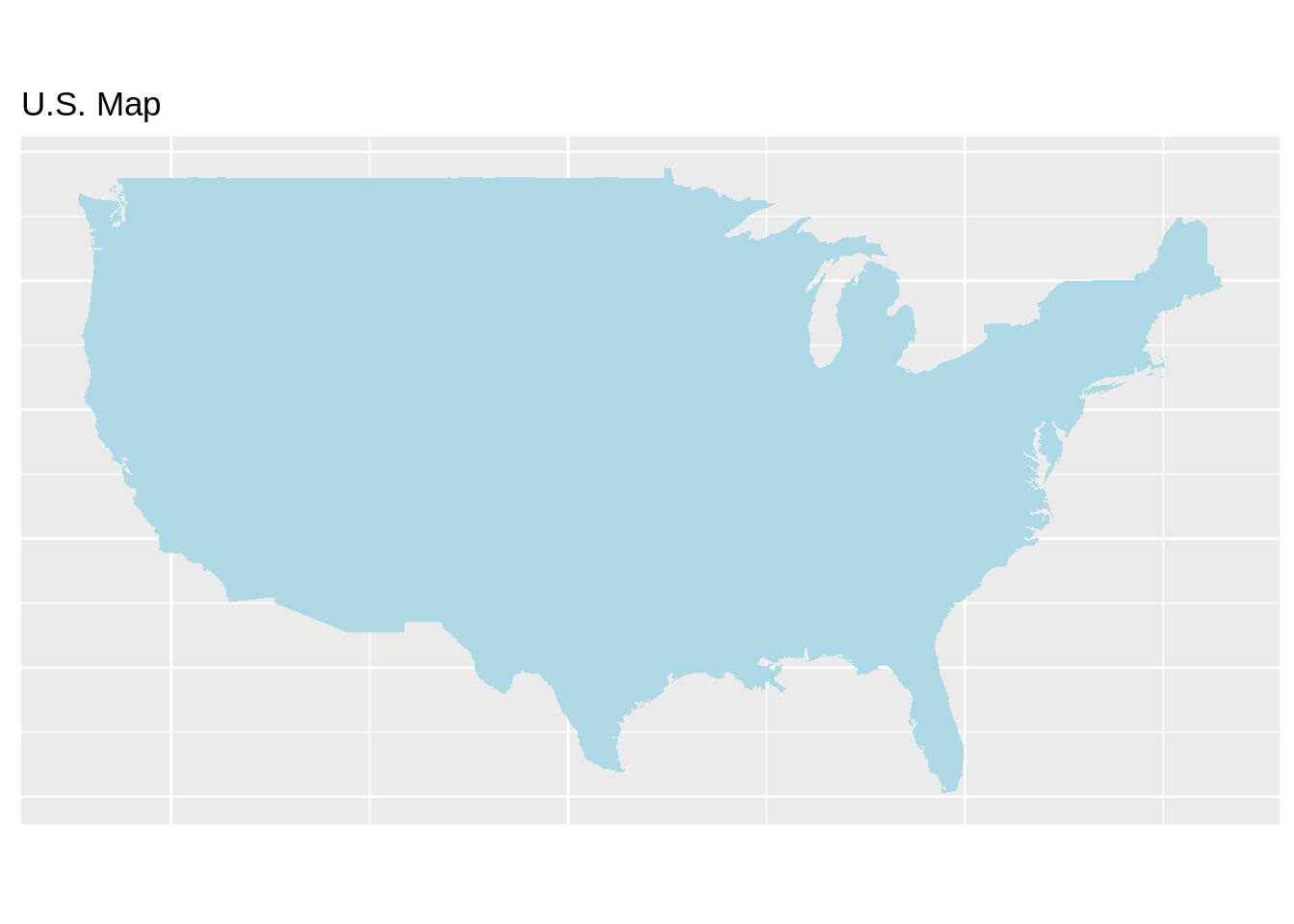In order to get more information about U.S., now we can add `state` data to the map. Like above, we obtain data from `maps` and `mapdata` packages, and use `ggplot2` to plot.

``state <- map_data("state")``
``````ggplot(data=state, aes(x=long, y=lat, fill=region, group=group)) +
geom_polygon(color = "white") +
guides(fill=FALSE) +
theme(axis.title.x=element_blank(), axis.text.x=element_blank(), axis.ticks.x=element_blank(),
axis.title.y=element_blank(), axis.text.y=element_blank(), axis.ticks.y=element_blank()) +
ggtitle('U.S. Map with States') +
coord_fixed(1.3)``````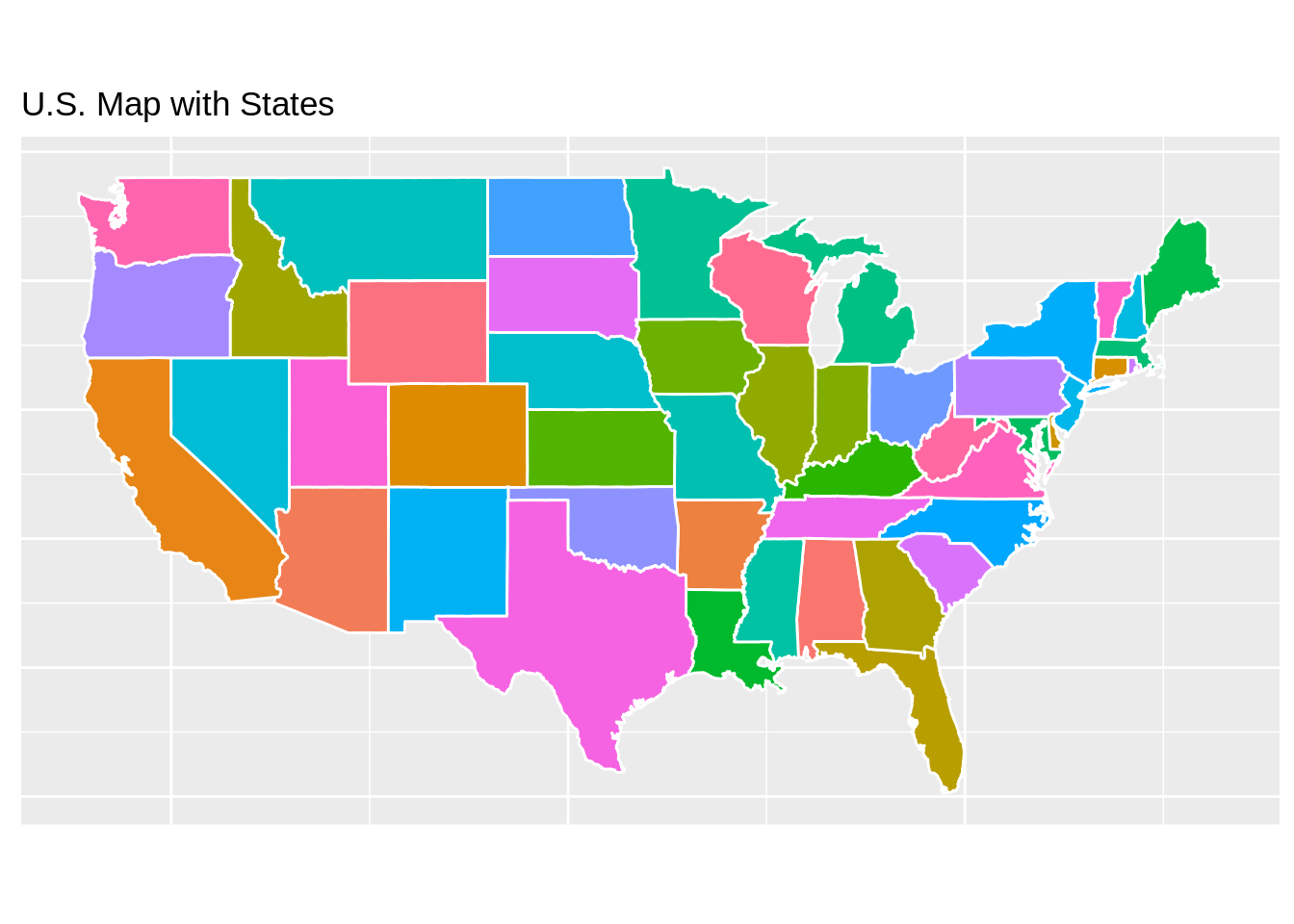What if we only care about one state? Definitely, we can draw one state map using `ggplot2`. Firstly we need to filter data.

``````washington <- subset(state, region=="washington")
counties <- map_data("county")
washington_county <- subset(counties, region=="washington")

ca_map <- ggplot(data=washington, mapping=aes(x=long, y=lat, group=group)) +
coord_fixed(1.3) +
geom_polygon(color="black", fill="gray") +
geom_polygon(data=washington_county, fill=NA, color="white") +
geom_polygon(color="black", fill=NA) +
ggtitle('Washington Map with Counties') +
theme(axis.title.x=element_blank(), axis.text.x=element_blank(), axis.ticks.x=element_blank(),
axis.title.y=element_blank(), axis.text.y=element_blank(), axis.ticks.y=element_blank())
ca_map``````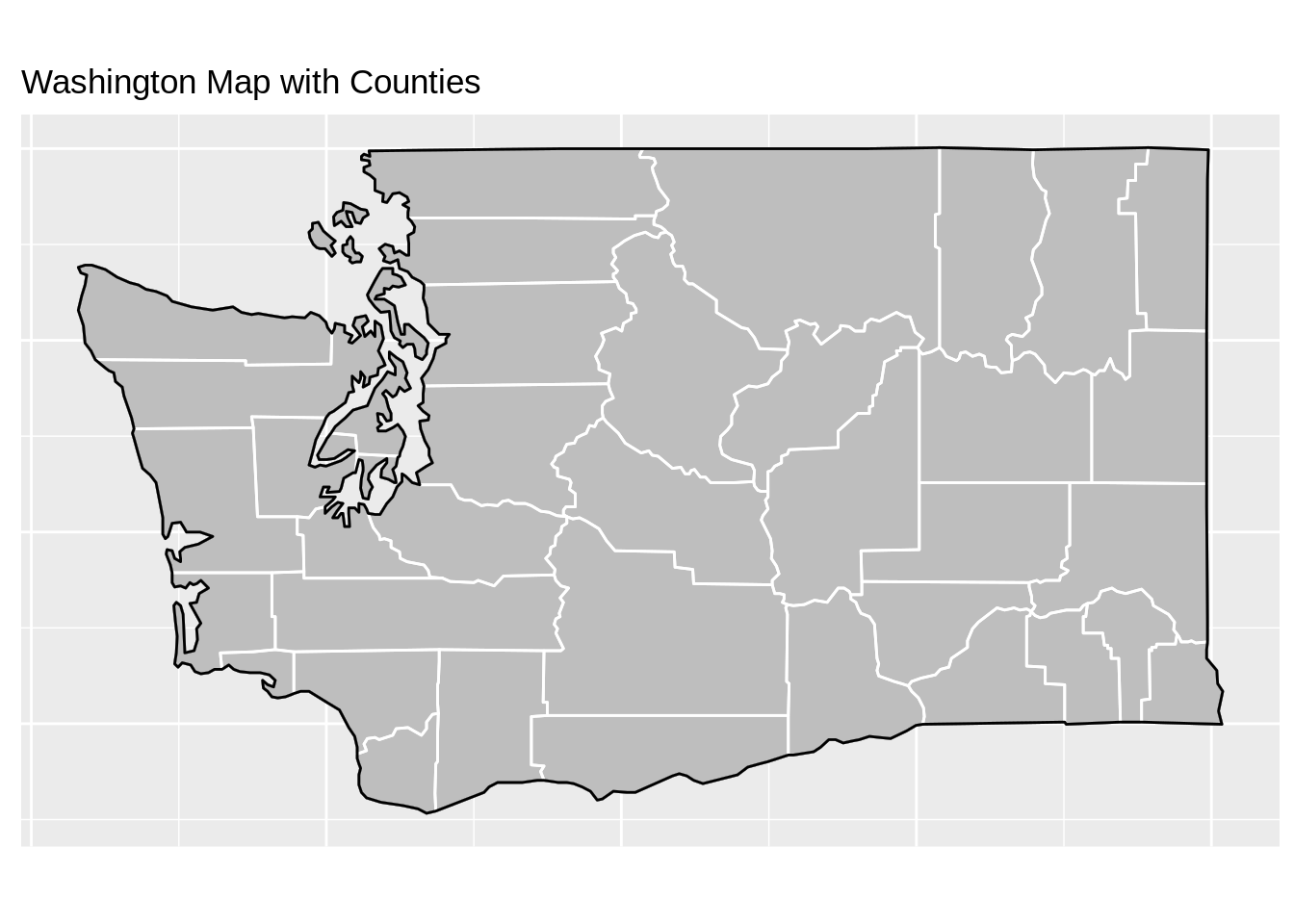## 41.4 Using `maps` package

(Note: In this part, we only care about continental U.S.)

We also can directly plot using `maps` package. However, with only `maps` package, we are limited by mapping techniques. Thus, we recommend using other packages instead.

Package(s) we need:

``````#install.packages("maps")
library(maps)``````
``````maps::map("state", interior=FALSE)
maps::map("state", boundary=FALSE, col="gray", add=TRUE)``````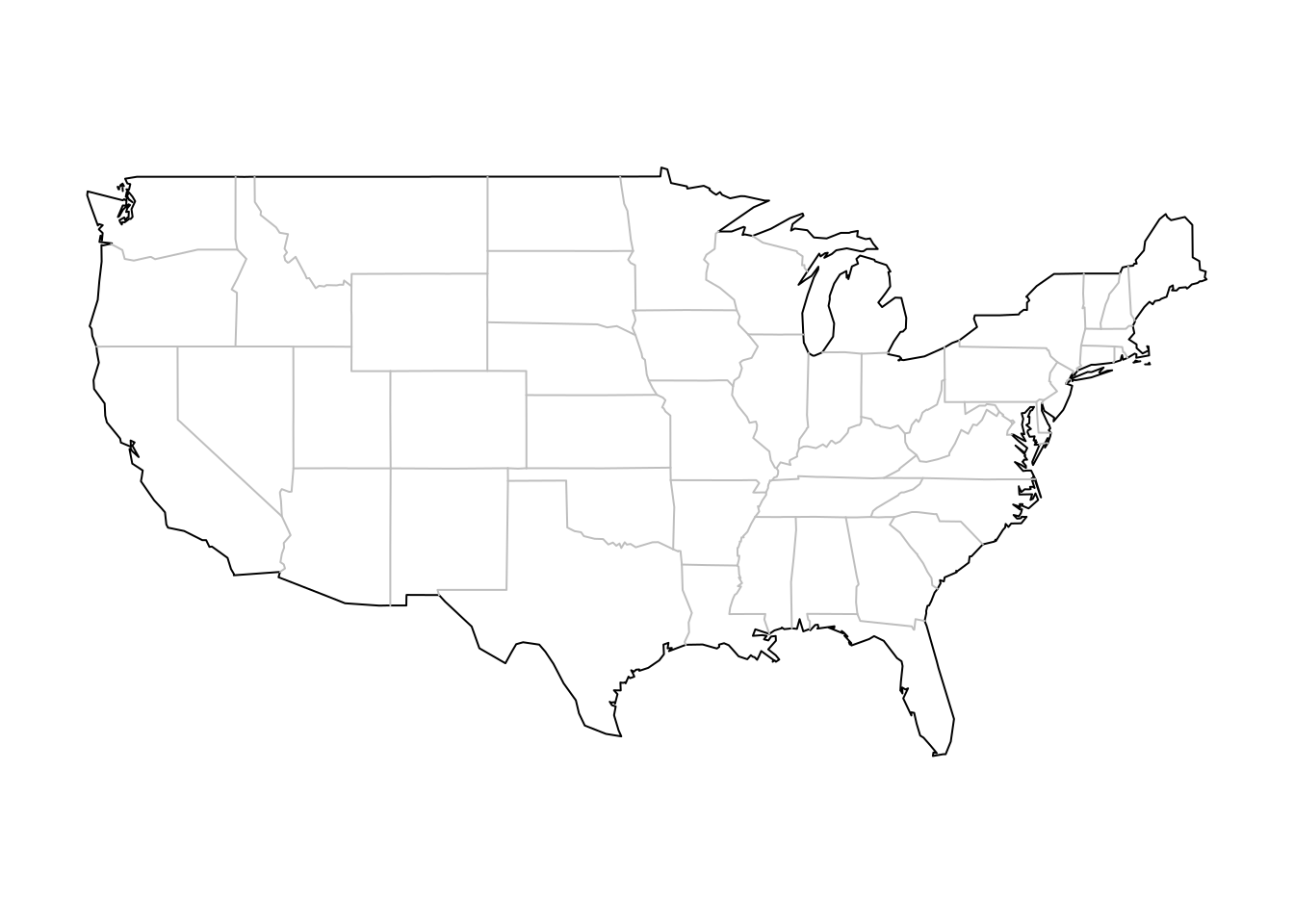## 41.5 Using `plotly` package

We first need to import data from `US-State-Boundaries-Census-2014.shp`, and then use `plotly` package. Suppose we care about water level for each state in U.S.

Package(s) we need:

``````library(tidyverse)
library(sf)
library(plotly)``````
``````states <- read_sf("resources/us_map/US-State-Boundaries-Census-2014.shp") %>%
st_zm() %>%
mutate(water_km2 = (AWATER / (1000*1000)) %>% round(2))``````
``````g <- ggplot(states) +
geom_sf(aes(fill=water_km2)) +
scale_fill_distiller("water level", palette="Spectral") +
ggtitle("Water by State")
ggplotly(g)``````

## 41.6 Using `mapview` package

We use the same dataset from last method. It gives us an interactive map with all information.

Package(s) we need:

``````#install.packages("mapview")
library(mapview)``````
``mapview(states)``

## 41.7 Using `leaflet` package

We use the same dataset from last method. We construct a interactive map with no specific information. If you are interested in a specific area, for example, water level, you can add specific choropleth to show more information.

Package(s) we need:

``````#install.packages("leaflet")
library(leaflet)``````
``````leaflet(states) %>%